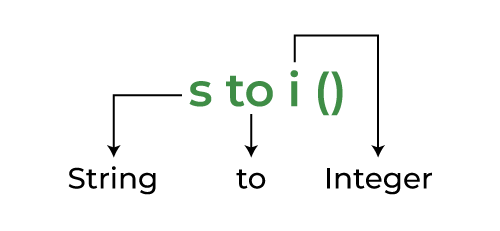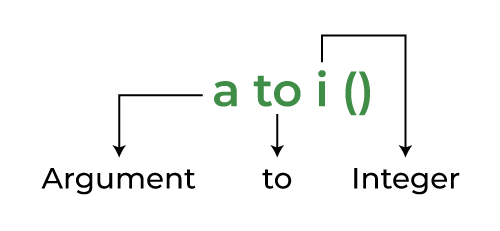GeeksforGeeks App
Open AppBrowser
Continue

# Convert String to int in C++

Converting a string to int is one of the most frequently encountered task in C++. As both string and int are not in the same object hierarchy, we cannot perform implicit or explicit type casting as we can do in case of double to int or float to int conversion. Conversion is mostly done so that we can convert numbers that are stored as strings.

Example:

str=”191″

num=191

There are 5 significant methods to convert strings to numbers in C++ as follows:

1. Using stoi() function
2. Using atoi() function
3. Using stringstream
4. Using sscanf() function
5. Using for Loop
6. Using strtol() function

## 1. String to int Conversion Using stoi() Function

The stoi() function in C++ takes a string as an argument and returns its value in integer form. This approach is popular in current versions of C++, as it was first introduced in C++11.

If you observe stoi() a little closer you will find out that it stands for:Breakdown of stoi() in simple terms

Syntax:

`int stoi(string str, size_t position = 0, int base = 10);`

Parameters:

• str: string to be converted. (compulsory)
• position: starting position. (optional with default value = 0)
• base: base of the number system. (optional with default value = 10)

Example:

## C++

 `// C++ program to demonstrate``// working of stoi()``// Work only if compiler supports``// C++11 or above. Because STOI()``// was added in C++ after 2011``#include ``#include ``using` `namespace` `std;` `// Driver code``int` `main()``{``  ``string str1 = ``"45"``;``  ``string str2 = ``"3.14159"``;``  ``char` `str3[] = ``"31337 geek"``;` `  ``// type of explicit type casting``  ``int` `myint1 = stoi(str1);``  ` `  ``// type of explicit type casting``  ``int` `myint2 = stoi(str2);``    ` `  ``// type of explicit type casting``  ``int` `myint3 = stoi(str3);` `  ``cout << ``"stoi(\""` `<< str1 <<``          ``"\") is "` `<< myint1 << ``'\n'``;``    ``cout << ``"stoi(\""` `<< str2 <<``            ``"\") is "` `<< myint2 << ``'\n'``;``    ``cout << ``"stoi(\""` `<< str3 <<``            ``"\") is "` `<< myint3 << ``'\n'``;``    ``return` `0;``}`

Output

```stoi("45") is 45
stoi("3.14159") is 3
stoi("31337 geek") is 31337```

As we can see, stoi() method supports both C++ style and C style strings.

We can also use atoi() function for performing this task.

## 2. String to int Conversion Using atoi()

The atoi() function in C++ takes a character array or string literal as an argument and returns its value in an integer. It is defined in the <stdlib.h> header file. This function is inherited by C++ from C language so it only works on C style strings i.e. array of characters.

If you observe atoi() a little closer you will find out that it stands for:Breakdown of atoi() in simple terms

Example:

## C++

 `// C++ program to demonstrate the``// functioning of the atoi() function``#include ``#include ``using` `namespace` `std;` `int` `main()``{``    ``char``* str1 = ``"141"``;``    ``char``* str2 = ``"3.14"``;` `    ``int` `res1 = ``atoi``(str1);``    ``int` `res2 = ``atoi``(str2);` `    ``cout << ``"atoi("` `<< str1 << ``") is "` `<< res1 << ``"\n"``;``    ``cout << ``"atoi("` `<< str2 << ``") is "` `<< res2 << ``"\n"``;` `    ``return` `0;``}`

Output

```atoi(141) is 141
atoi(3.14) is 3 ```

## 3. String to int Conversion Using stringstream Class

The stringstream class in C++  allows us to associate a string to be read as if it were a stream. We can use it to easily convert strings of digits into ints, floats, or doubles. The stringstream class is defined inside the <sstream> header file.

It works similar to other input and output streams in C++. We first create a stringstream object as shown below:

Syntax:

`stringstream ss;`

Then insert a numeric string into it using the ( << ) insertion operator.

Example:

```ss << myString;
ss << myCstring;
ss << myInt; or float, or double, etc.```

At last, we extract the numeric value from the stream using the ( >> ) extraction operator.

Example:

```ss >> myChar;
ss >> myCstring;
ss >> myInt;```

The below C++ program demonstrates how to convert a string to int using a stringstream object:

## C++

 `// C++ program to demonstrate the``// use of a stringstream to``// convert string to int``#include ``#include ``using` `namespace` `std;` `// Driver code``int` `main()``{``    ``string s = ``"12345"``;` `    ``// object from the class stringstream``    ``stringstream geek;` `    ``// inserting string s in geek stream``    ``geek << s;` `    ``// The object has the value 12345``    ``// and stream it to the integer x``    ``int` `x = 0;``    ``geek >> x;` `    ``// Now the variable x holds the``    ``// value 12345``    ``cout << ``"Value of x + 1 : "` `<< x + 1;` `    ``return` `0;``}`

Output

`Value of x + 1 : 12346`

To summarize, stringstream is a convenient way to manipulate strings. This method also works for both C style strings or C++ style strings.

## 4. Converting String to int Using sscanf()

‘sscanf() is a C-style function similar to scanf(). It reads input from a string rather than standard input.

Syntax of sscanf:

`int sscanf (const char * source, const char * formatted_string, ...);`

Parameters:

• source  –  source string.
• formatted_string  –  a string that contains the format specifiers.
• … :  –  variable arguments list that contains the address of the variables in which we want to store input data.

There should be at least as many of these arguments as the number of values stored by the format specifiers.

Return:

• On success, the function returns the number of variables filled.
• In the case of an input failure, before any data could be successfully read, the end of the function(EOF) is returned.

Example:

## C++

 `// C++ program to demonstrate``// the working of sscanf() to``// convert a string into a number``#include ``using` `namespace` `std;` `int` `main()``{``    ``const` `char``* str = ``"12345.0000046"``;``    ``float` `x;``    ``sscanf``(str, ``"%f"``, &x);` `    ``cout << ``"The value of x : "` `<< x << endl;``    ``return` `0;``}`

Output

`The value of x : 12345`

## 5. Using For Loop Convert Strings into int

It is the naive method of string-to-int conversion where we compare each character to its ASCII values and then get the value of that numeric character.

Below is the C++ program used for loop to convert strings into numbers:

## C++

 `// C++ Program to convert String``// into number using for loop``#include ` `using` `namespace` `std;``int` `main()``{``    ``string number = ``"13"``;``    ``int` `i = 0;` `    ``// Traversing string``    ``for` `(``char` `c : number) {``        ``// Checking if the element is number``        ``if` `(c >= ``'0'` `&& c <= ``'9'``) {``            ``i = i * 10 + (c - ``'0'``);``        ``}``        ``// Otherwise print bad output``        ``else` `{``            ``cout << ``"Bad Input"``;``            ``return` `1;``        ``}``    ``}` `    ``cout << i;``}`

Output

`13`

## 6. String to int Conversion Using strtol()

Using the strtol functions: It converts a string to a long integer value, respectively.

Syntax of strtol():

`long int strtol(const char* str, char** endptr, int base);`

Parameters:

• str- Pointer to the null-terminated byte string to be converted
• endptr- Reference to a pointer to character. The function stores the address of the first invalid character in *endptr. If endptr is a null pointer, the function does not store this information.
• base- The number base to interpret the string. Must be between 2 and 36, inclusive, or 0. If base is 0, the function determines the base from the string itself. If the base is 16, the string may begin with the prefix “0x” or “0X”, in which case the number is parsed as a hexadecimal value.

Return:

• If the string is empty or does not contain any digits, 0 is returned.
• If the conversion is successful, the function returns the converted long integer value.
• If the conversion fails, the function returns 0 and sets *endptr to point to the beginning of the string.

Example:

## C++

 `#include ``#include ` `int` `main() {``  ``char` `str[] = ``"12345"``;``  ``char``* endptr;``  ``long` `int` `value = ``strtol``(str, &endptr, 10);``  ``if` `(endptr == str) {``    ``printf``(``"No digits were found\n"``);``  ``} ``else` `if` `(*endptr != ``'\0'``) {``    ``printf``(``"Invalid input: %s\n"``, str);``  ``} ``else` `{``    ``printf``(``"The value is %ld\n"``, value);``  ``}``  ``return` `0;``}`

Output

`The value is 12345`

Time Complexity: O(N), where N is the number of characters in the string.
Auxiliary Space: O(1)

My Personal Notes arrow_drop_up